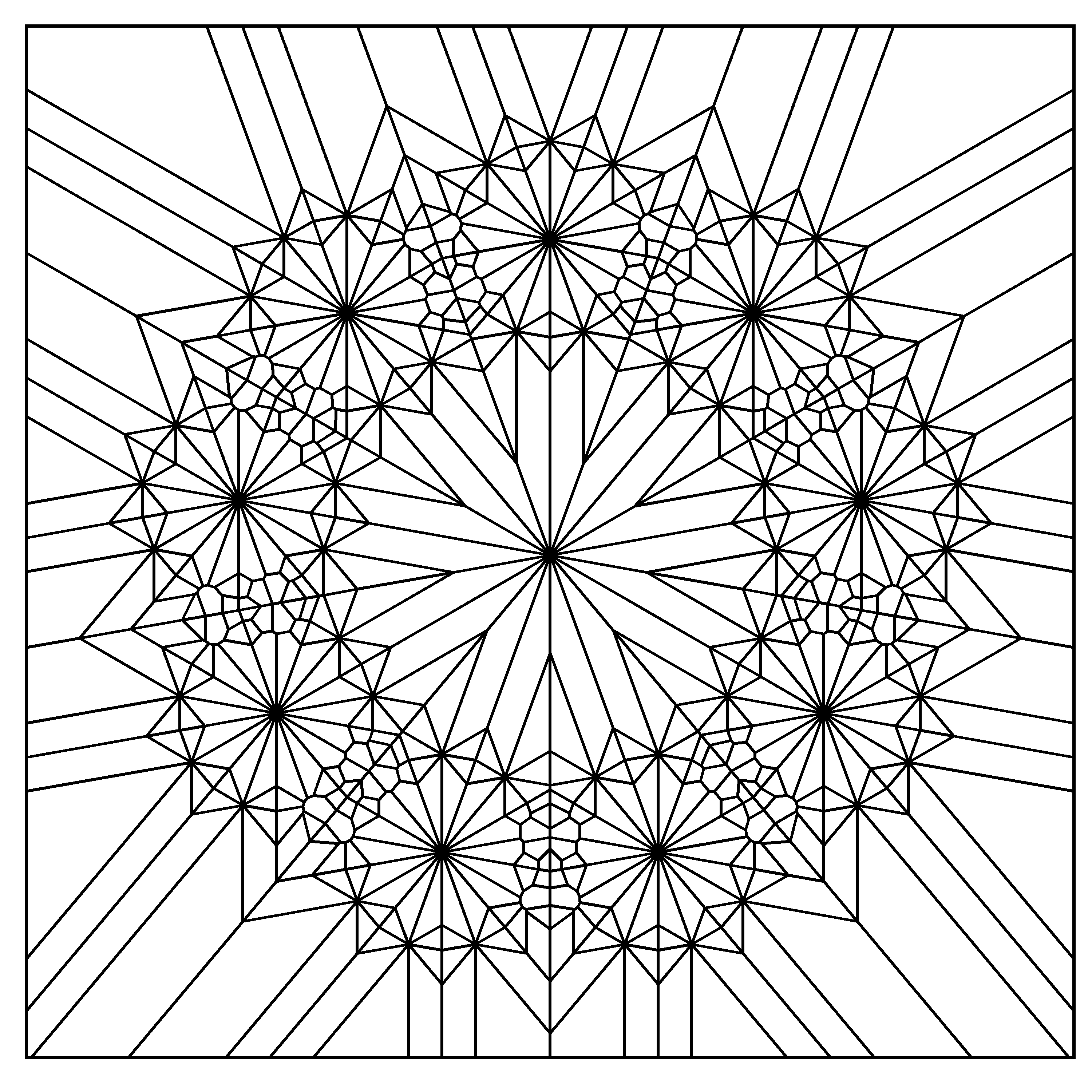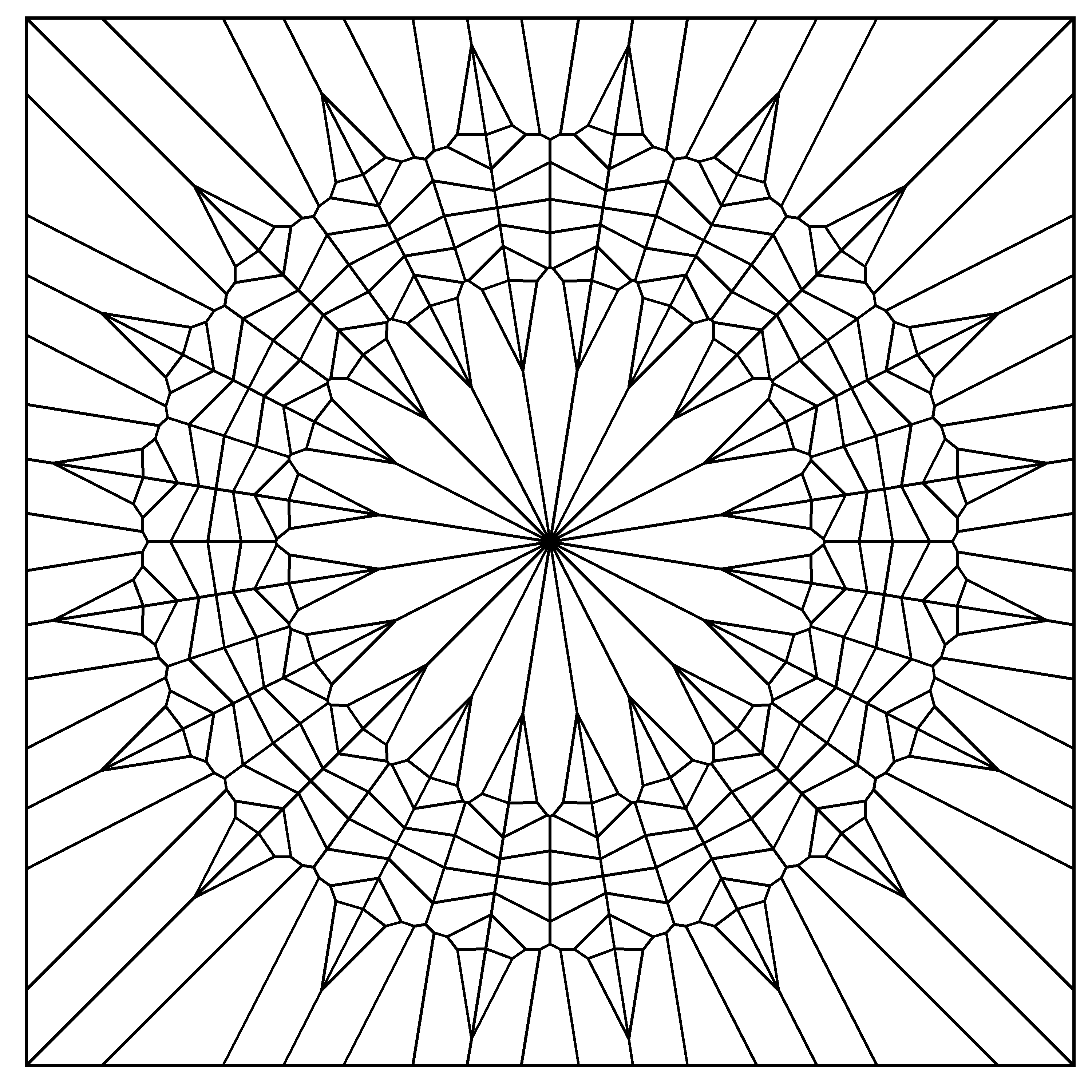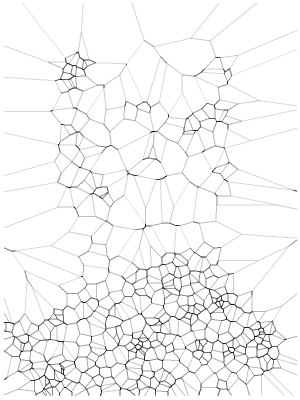# Mandalas Colored

Apriétame bien la mano, que un lucero se me escapa entre los dedos (Coda Flamenca, Extremoduro)

I have the privilege of being teacher at ESTALMAT, a project run by Spanish Royal Academy of Sciences that tries to detect, guide and stimulate in a continuous way, along two courses, the exceptional mathematical talent of students of 12-13 years old. Some weeks ago I gave a class there about the importance of programming. I tried to convince them that learning R or Python is a good investment that always pays off; It will make them enjoy more of mathematics as well as to see things with their own eyes. The main part of my class was a workshop about Voronoi tesselations in R. We started drawing points on a circle and we finished drawing mandalas like these ones. You can find the details of the workshop here (in Spanish). It was a wonderful experience to see the faces of the students while generating their own mandalas.

In that case all mandalas were empty, ready to be printed and coloured as my 7 years old daughter does. In this experiment I colour them. These are the changes I have done to my  previous code:

• Remove external segments which intersects the boundary of the enclosing
rectangle
• Convert the tesselation into a list of polygons with `tile.list` function
• Use `colourlovers` package to fill the polygons with beautiful colour palettes

This is an example of the result:Changing three simple parameters (`iter`, `points` and `radius`) you can obtain completely different images (clicking on any image you can see its full size version):

You can find details of these parameters in my previous post. I cannot resist to place more examples:

You can find the code here. Enjoy.

# Mandalas

Mathematics is a place where you can do things which you can’t do in the real world (Marcus Du Sautoy, mathematician)

From time to time I have a look to some of my previous posts: it’s like seeing them through another’s eyes. One of my first posts was this one, where I draw fractals using the Multiple Reduction Copy Machine (MRCM) algorithm. That time I was not clever enough to write an efficient code able generate deep fractals. Now I am pretty sure I could do it using `ggplot` and I started to do it when I come across with the idea of mixing this kind of fractal patterns with Voronoi tessellations, that I have explored in some of my previous posts, like this one. Mixing both techniques, the mandalas appeared.

I will not explain in depth the mathematics behind this patterns. I will just give a brief explanation:

• I start obtaining `n` equidistant points in a unit circle centered in `(0,0)`
• I repeat the process with all these points, obtaining again `n` points around each of them; the radius is scaled by a factor
• I discard the previous (parent) `n` points

I repeat these steps iteratively. If I start with n points and iterate k times, at the end I obtain nk points. After that, I calculate the Voronoi tesselation of them, which I represent with `ggplot`.

This is an example:Some others:You can find the code here. Enjoy it.

# Frankenstein

Remember me, remember me, but ah! forget my fate (Dido’s Lament, Henry Purcell)

A Voronoi diagram divides a plane based on a set of original points. Each polygon, or Voronoi cell, contains an original point and all that are closer to that point than any other.

This is a nice example of a Voronoi tesselation. You can find good explanations of Voronoi diagrams and Delaunay triangulations here (in English) or here (in Spanish).

A grayscale image is simply a matrix where darkness of pixel located in coordinates (i, j) is represented by the value of its corresponding element of the matrix: a grayscale image is a dataset. This is a Voronoi diagraman of Frankenstein:To do it I followed the next steps:

1. Read this image
2. Convert it to gray scale
3. Turn it into a pure black and white image
4. Obtain a random sample of black pixels (previous image corresponds to a sample of 6.000 points)
5. Computes the Voronoi tesselation

Steps 1 to 3 were done with imager, a very appealing package to proccess and analice images. Step 5 was done with deldir, also a convenient package which computes Delaunay triangulation and the Dirichlet or Voronoi tessellations.

The next grid shows tesselations for sample size from 500 to 12.000 points and step equal to 500:

I gathered all previous images in this gif created with magick, another amazing package of R I discovered recently:This is the code:

```library(imager)
library(dplyr)
library(deldir)
library(ggplot2)
library(scales)

download.file(file, destfile = "frankenstein.jpg", mode = 'wb')

# Read and convert to grayscale
load.image("frankenstein.jpg") %>% grayscale() -> x

# This is just to define frame limits
x %>%
as.data.frame() %>%
group_by() %>%
summarize(xmin=min(x), xmax=max(x), ymin=min(y), ymax=max(y)) %>%
as.vector()->rw

# Filter image to convert it to bw
x %>%
threshold("45%") %>%
as.cimg() %>%
as.data.frame() -> df

# Function to compute and plot Voronoi tesselation depending on sample size
doPlot = function(n)
{
#Voronoi tesselation
df %>%
sample_n(n, weight=(1-value)) %>%
select(x,y) %>%
deldir(rw=rw, sort=TRUE) %>%
.\$dirsgs -> data

# This is just to add some alpha to lines depending on its longitude
data %>%
mutate(long=sqrt((x1-x2)^2+(y1-y2)^2),
alpha=findInterval(long, quantile(long, probs = seq(0, 1, length.out = 20)))/21)-> data

# A little bit of ggplot to plot results
data %>%
ggplot(aes(alpha=(1-alpha))) +
geom_segment(aes(x = x1, y = y1, xend = x2, yend = y2), color="black", lwd=1) +
scale_x_continuous(expand=c(0,0))+
scale_y_continuous(expand=c(0,0), trans=reverse_trans())+
theme(legend.position  = "none",
panel.background = element_rect(fill="white"),
axis.ticks       = element_blank(),
panel.grid       = element_blank(),
axis.title       = element_blank(),
axis.text        = element_blank())->plot

return(plot)
}

# I call the previous function and store resulting plot in jpeg format
i=5000
name=paste0("frankie",i,".jpeg")
jpeg(name, width = 600, height = 800, units = "px", quality = 100)
doPlot(i)
dev.off()

# Once all images are stored I can create gif
library(magick)
frames=c()
images=list.files(pattern="jpeg")

for (i in length(images):1)
{